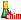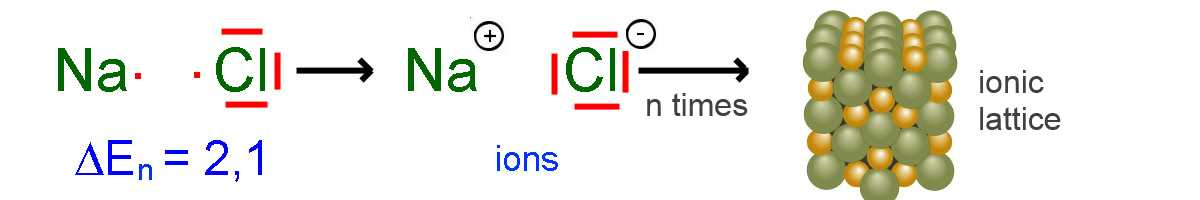Search:

# Electronegativity and the chemical bonds

→    Table of electronegativities

## Principle

Atoms with a small electronegativity abandon easily their outer electrons, atoms with a big electronegativity catch easily foreign electrons.

## Electronegativity and the ionic bond

If the difference of the electronegativities of two atoms exceeds 2, an ionic bond is made.

For example, $E.n.(Cl)-E.n.(Na) = 2.1$, so sodium chloride is an ionic substance.## Electronegativity and the covalent bond

Two atoms with electronegativities greater than or equal to 2 combine to a covalent bond.

For example, $E.n.(H), E.n.(O) > 2$, so water is a molecular substance.## Electronegatvity and the metallic bond

Atoms with electronegativities less than 2 combine to metallic bonds.

For example, $E.n.(Na)=0.9 2$, therefore is sodium a metal.## Comment

The limit of 2 that we have given ourselves is vague. Near to this limit, intermediate cases are all to common, for example, - in a sample of iron(III)chloride $(\Delta(E.n.)$ $=$ $1.2)$ there are molecules of $FeCl_3$, but also $Fe^{3+}$ and $Cl^-$ ions! - gold ($E.n.=2.4$) is a metal!